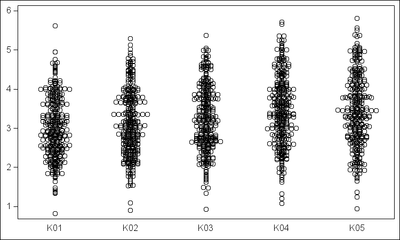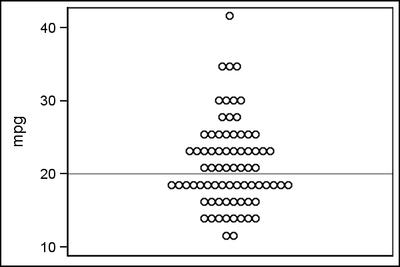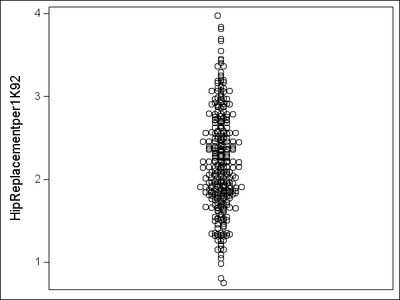## running Turnip chart demo code

SAS UniversityEdition

This is the log file when I run the Turnip Chart demo: Can SAS UniversityEdition runn this code and how?

1          OPTIONS NONOTES NOSTIMER NOSOURCE NOSYNTAXCHECK;
NOTE: GOPTIONS statements in the SAS Studio environment may disable some output features.
NOTE: ODS statements in the SAS Studio environment may disable some output features.
57
58         %let name=turnip;
59         filename odsout '.';
60
61         /* Get your data into a sas data set.
62            If you use these exact same variable names, you can re-use
63            this exact same sas code.  If this code is converted into
64            a generalized macro, the variable names could be parameters
65            that are passed in.
66         */
67         data mydata;
68         format percent percentn9.2;
69         format cum_percent percentn9.2;
70         input mpg freq percent cum_percent;
71         percent=percent/100;
72         cum_percent=cum_percent/100;
73         datalines;

NOTE: The data set WORK.MYDATA has 11 observations and 4 variables.
NOTE: DATA statement used (Total process time):
real time           0.00 seconds
cpu time            0.01 seconds

85         ;

86         run;
87
88         /* Create one plot marker for each 'freq', and position it symmetrically
89            around x=0.  Note that these are centered differently for odd-versus-even
90            numbers of markers. */
91         data plotdata; set mydata;
92         /* Even number of markers */
93         if (freq/2) eq int(freq/2) then do;
94          do lcv=1 to (freq/2);
95           x=lcv-.5; output;
96           x=-1*(lcv-.5); output;
97          end;
98         end;
99         /* Odd number of markers */
100        else do;
101         do lcv=1 to ((freq+1)/2);
102          x=lcv-1; output;
103          x=-1*(lcv-1); output;
104         end;
105        end;
106        run;

NOTE: There were 11 observations read from the data set WORK.MYDATA.
NOTE: The data set WORK.PLOTDATA has 78 observations and 6 variables.
NOTE: DATA statement used (Total process time):
real time           0.00 seconds
cpu time            0.01 seconds

107
108        goptions device=png;
108        goptions device=png;
________
180
ERROR 180-322: Statement is not valid or it is used out of proper order.

109        goptions noborder;
109        goptions noborder;
________
180
ERROR 180-322: Statement is not valid or it is used out of proper order.

110
111        ODS LISTING CLOSE;
112        ODS HTML path=odsout body="&name..htm" (title="Custom SAS/Graph Turnip Chart") style=htmlblue;
NOTE: Writing HTML Body file: turnip.htm
ERROR: Insufficient authorization to access /opt/sasinside/SASConfig/Lev1/SASApp/turnip.htm.
ERROR: No body file. HTML output will not be created.
113
114        goptions gunit=pct htitle=5 ftitle="albany amt/bold" htext=3 ftext="albany amt";
114        goptions gunit=pct htitle=5 ftitle="albany amt/bold" htext=3 ftext="albany amt";
________
180
ERROR 180-322: Statement is not valid or it is used out of proper order.

115
116        symbol1 value=circle height=4 interpol=none color=black;
116        symbol1 value=circle height=4 interpol=none color=black;
_______
180
ERROR 180-322: Statement is not valid or it is used out of proper order.

117
118        axis1 label=(angle=90) order=(9 to 45 by 36) minor=none offset=(5,5) reflabel=("20");
118        axis1 label=(angle=90) order=(9 to 45 by 36) minor=none offset=(5,5) reflabel=("20");
_____
180
ERROR 180-322: Statement is not valid or it is used out of proper order.

119
120        axis2 label=none order=(-16 to 16 by 32) minor=(number=1) offset=(5,5);
120        axis2 label=none order=(-16 to 16 by 32) minor=(number=1) offset=(5,5);
_____
180
ERROR 180-322: Statement is not valid or it is used out of proper order.

121
122        title1 ls=1.5 link="turnip_info.htm" "Turnip Chart";
123
124        proc gplot data=plotdata;
125        plot mpg*x=1 / noframe
126         vaxis=axis1 haxis=axis2
127         vref=20 cvref=gray
128         des='' name="&name" ;
129        run;

NOTE: The SAS System stopped processing this step because of errors.
NOTE: PROCEDURE GPLOT used (Total process time):
real time           0.00 seconds
cpu time            0.00 seconds

130

131        proc print data=mydata noobs;
132        var mpg freq percent cum_percent;
133        run;

NOTE: There were 11 observations read from the data set WORK.MYDATA.
NOTE: PROCEDURE PRINT used (Total process time):
real time           0.03 seconds
cpu time            0.04 seconds

134
135        quit;
136        ODS HTML CLOSE;
137        ODS LISTING;
138
139        OPTIONS NONOTES NOSTIMER NOSOURCE NOSYNTAXCHECK;
151

User: sasdemo
1 ACCEPTED SOLUTION

Accepted SolutionsJay54
Meteorite | Level 14

## Re: running Turnip chart adapting demo code to my sas data set and variables

Here is a graph with program.  You could restructure your data by category (each of the "Kxx" columns for easier processing.  Anyway, I kept your data structure, and just added some columns with the last 3 characters of each response variable as the category, such as "K01", "K02", etc, and used them with the corresponding response variable.``````%let gpath='.';
%let dpi=150;
ods html close;
ods listing image_dpi=&dpi gpath=&gpath;

proc import datafile="C:\qry_hip.xlsx"
dbms=xlsx out=Hip_Replacement replace;
run;

data hip;
set hip_replacement;
K01='K01'; K02='K02'; K03='K03'; K04='K04'; K05='K05';
run;

/*--Plot Graph--*/
ods graphics / reset width=5in height=3in imagename='Turnip_Hip';
proc sgplot data=hip nocycleattrs noautolegend;
scatter x=k01 y=HipReplacementper1K01 / jitter;
scatter x=k02 y=HipReplacementper1K02 / jitter;
scatter x=k03 y=HipReplacementper1K03 / jitter;
scatter x=k04 y=HipReplacementper1K04 / jitter;
scatter x=k05 y=HipReplacementper1K05 / jitter;
xaxis display=(noticks nolabel);
yaxis display=(nolabel);
run;
``````

(Editor's note: Full example of a scalable Turnip graph is available on this blog post.)

28 REPLIES 28

## Re: running Turnip chart demo code

GOPTIONS is related to settings for the traditional device based graphics found in SAS/GRAPH  which is not part of University edition.

Look at the ODS GRAPHICS statement to set options such as IMAGEFM. But PNG will generally be the default for graphics so you may not need to worry.Jay54
Meteorite | Level 14

## Re: running Turnip chart demo code

As noted by "ballardW", SAS/GRAPH is not included with SAS University Edition.  But Base Graphics procedures are included such as  SGPLOT. Reviewing Robert's example, it looks like a "Jittered" scatter plot.  Should be doable using SGPLOT.Jay54
Meteorite | Level 14

## Re: running Turnip chart demo code

``````%let gpath='.';
%let dpi=200;

data mydata;
format percent percentn9.2;
format cum_percent percentn9.2;
input mpg freq percent cum_percent;
percent=percent/100;
cum_percent=cum_percent/100;
Cat='A';
datalines;
11.57101  2  2.70   2.70
13.88521  8 10.81  13.51
16.19941  8 10.81  24.32
18.51361 17 22.97  47.30
20.82781  8 10.81  58.11
23.14201 12 16.22  74.32
25.45621  8 10.81  85.14
27.77042  3  4.05  89.19
30.08462  4  5.41  94.59
34.71302  3  4.05  98.65
41.65562  1  1.35 100.00
;
run;

/*--Expand the data--*/
data expand;
set mydata;
do i=1 to freq;
output;
end;
run;

/*--Draw Graph--*/
title;
ods html close;
ods listing gpath=&gpath image_dpi=&dpi;
ods graphics / reset width=3in height=2in imagename='Turnip';
proc sgplot data=expand;
scatter x=cat y=mpg / jitter;
refline 20;
xaxis display=(noticks novalues nolabel);
run;
``````## Re: running Turnip chart adapting demo code to my sas data set and variables

How do I adapt demo code to use my sas data set and variables

Can you suggest how I can produce turnip charts of variables in qry_hipReplacement1992to2013.xlsx

Greatly appreciative,

jemohr22

## Re: running Turnip chart adapting demo code to my sas data set and variablesJay54
Meteorite | Level 14

## Re: running Turnip chart adapting demo code to my sas data set and variables

Which variable from this data do you want to plot?  This is one of them.## Re: running Turnip chart adapting demo code to my sas data set and variables

The hipreplacement variable you chose is good. What does the sas code look like?

My professor asked me to produce a series of turnip graphs

Thank you

## Re: running Turnip chart adapting demo code to my sas data set and variables

I need to produce a series of Turnip Charts for all variables in this data set. It appears that you were able to successfully produce a Turnip Chart for one of the variables. Could you share the code? I assume that with the code I will be able to simply change the variable name

In an ideal world I would be able to display a Turnip Chart Graphic for multiple variables in the same output. See attached pdf fileJay54
Meteorite | Level 14

## Re: running Turnip chart adapting demo code to my sas data set and variables

Here is a graph with program.  You could restructure your data by category (each of the "Kxx" columns for easier processing.  Anyway, I kept your data structure, and just added some columns with the last 3 characters of each response variable as the category, such as "K01", "K02", etc, and used them with the corresponding response variable.``````%let gpath='.';
%let dpi=150;
ods html close;
ods listing image_dpi=&dpi gpath=&gpath;

proc import datafile="C:\qry_hip.xlsx"
dbms=xlsx out=Hip_Replacement replace;
run;

data hip;
set hip_replacement;
K01='K01'; K02='K02'; K03='K03'; K04='K04'; K05='K05';
run;

/*--Plot Graph--*/
ods graphics / reset width=5in height=3in imagename='Turnip_Hip';
proc sgplot data=hip nocycleattrs noautolegend;
scatter x=k01 y=HipReplacementper1K01 / jitter;
scatter x=k02 y=HipReplacementper1K02 / jitter;
scatter x=k03 y=HipReplacementper1K03 / jitter;
scatter x=k04 y=HipReplacementper1K04 / jitter;
scatter x=k05 y=HipReplacementper1K05 / jitter;
xaxis display=(noticks nolabel);
yaxis display=(nolabel);
run;
``````

(Editor's note: Full example of a scalable Turnip graph is available on this blog post.)

## Re: running Turnip chart adapting demo code to my sas data set and variables

Here is the code I ran. The excel file was located at C:\qry_hip.xlsx

%let gpath='.';
%let dpi=150;
ods html close;
ods listing image_dpi=&dpi gpath=&gpath;

proc import datafile="C:\qry_hip.xlsx"
dbms=xlsx out=Hip_Replacement replace;
run;

data hip;
set hip_replacement;
K01='K01'; K02='K02'; K03='K03'; K04='K04'; K05='K05';
run;

/*--Plot Graph--*/
ods graphics / reset width=5in height=3in imagename='Turnip_Hip';
proc sgplot data=hip nocycleattrs noautolegend;
scatter x=k01 y=HipReplacementper1K01 / jitter;
scatter x=k02 y=HipReplacementper1K02 / jitter;
scatter x=k03 y=HipReplacementper1K03 / jitter;
scatter x=k04 y=HipReplacementper1K04 / jitter;
scatter x=k05 y=HipReplacementper1K05 / jitter;
xaxis display=(noticks nolabel);
yaxis display=(nolabel);
run;

## Re: running Turnip chart adapting demo code to my sas data set and variables

It appears that some of the code in the first demo code example is missing from the code you sent along with the Turnip Chart depicting multiple years.

Instead of importing the qry_hips.xlsx could I reference a SAS data set created by importing the qry_hips.xlsx to create a SAS dataset WORK.QRY_HIPS92TO13

I have tried running the code you sent, but I get multiple error messages including that the physical file qry_hips.xlsx can not be found or does not exist

Could you please forward a copy of all code used to produce the multi year Turnip Chart.  I should be able to produce similar charts using the SAS University Edition by changing a few variable names

I am facing a publication deadlineJay54
Meteorite | Level 14

## Re: running Turnip chart adapting demo code to my sas data set and variables

"qry_hips.xlsx" is the same as your original xlsx file.  I used proc IMPORT to read the Excel data.  I don't know if UA has PROC IMPORT?  I attached the data set I imported in a zip file.  You can try using that.

## Re: running Turnip chart adapting demo code to my sas data set and variables

I appreciate the value of your time and would be grateful if you could guide me to the point of having a SAS program that produces a turnip chart for my HLTHDATA.HIPS9213 SAS dat set.

I imported qry_hips.xlsx into a SAS data set into a library, HLTHDATA.HIPS9213. The attached pdf file is the output of proc contents on HLTHDATA.HIPS9213. It lists the variable names I will need to use. I should be able to access this SAS data set using the data step:

data WORK.HIPREP;

set HLTHDATA.HIPS9213;

run;

What follows this data step? Where is the code that functions as the following sections of the original demo code?

data mydata;
format percent percentn9.2;
format cum_percent percentn9.2;
input mpg freq percent cum_percent;
percent=percent/100;
cum_percent=cum_percent/100;
Cat='A';
datalines;
11.57101  2  2.70   2.70
13.88521  8 10.81  13.51
16.19941  8 10.81  24.32
18.51361 17 22.97  47.30
20.82781  8 10.81  58.11
23.14201 12 16.22  74.32
25.45621  8 10.81  85.14
27.77042  3  4.05  89.19
30.08462  4  5.41  94.59
34.71302  3  4.05  98.65
41.65562  1  1.35 100.00
;
run;

/*--Expand the data--*/
data expand;
set mydata;
do i=1 to freq;
output;
end;
run;

/*--Draw Graph--*/
title;
ods html close;
ods listing gpath=&gpath image_dpi=&dpi;
ods graphics / reset width=7in height=7in imagename='Turnip';
proc sgplot data=expand;
scatter x=cat y=mpg / jitter;
refline 20;
xaxis display=(noticks novalues nolabel);
run;

## Re: running Turnip chart adapting demo code to my sas data set and variables

Thank you so very much!

I was able to make it work.

Now I will tweak refinements to the display

Discussion stats
• 28 replies
• 2743 views
• 0 likes
• 4 in conversation